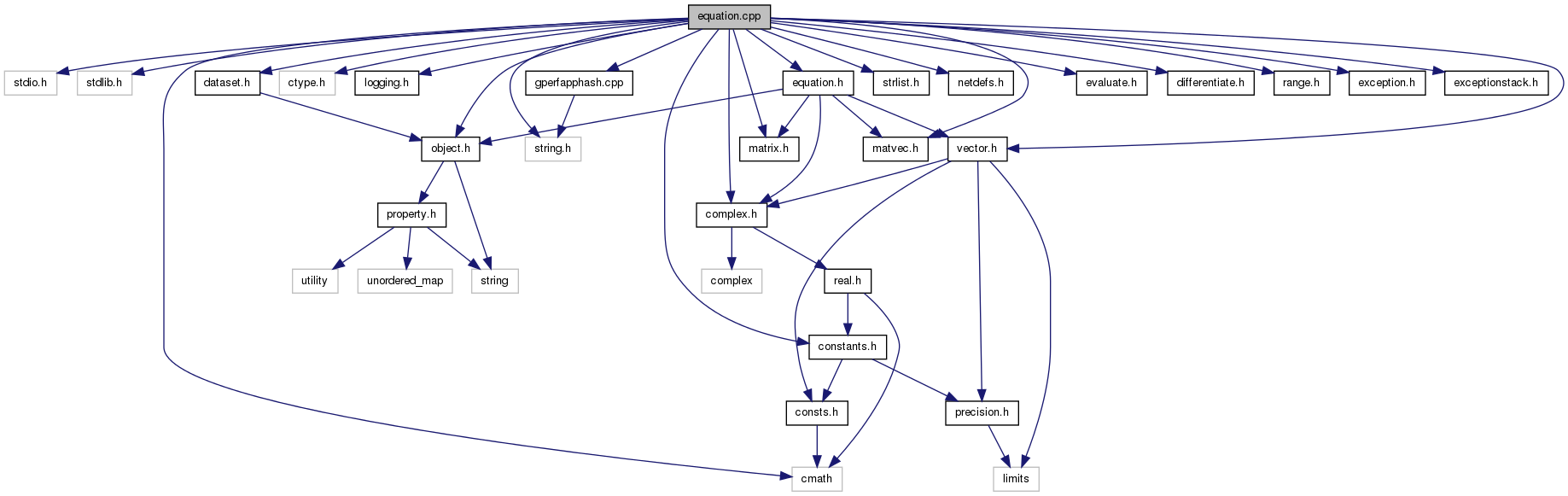Qucs-core  0.0.19
equation.cpp File Reference
`#include <stdio.h>`
`#include <stdlib.h>`
`#include <string.h>`
`#include <cmath>`
`#include <ctype.h>`
`#include "logging.h"`
`#include "complex.h"`
`#include "object.h"`
`#include "vector.h"`
`#include "matrix.h"`
`#include "matvec.h"`
`#include "dataset.h"`
`#include "strlist.h"`
`#include "netdefs.h"`
`#include "equation.h"`
`#include "evaluate.h"`
`#include "differentiate.h"`
`#include "constants.h"`
`#include "range.h"`
`#include "exception.h"`
`#include "exceptionstack.h"`
`#include "gperfapphash.cpp"`
Include dependency graph for equation.cpp:Go to the source code of this file.

## Data Structures

struct  qucs::appindex
class  qucs::gperfapphash
struct  qucs::pconstant

namespace  qucs

## Defines

#define A(a)   ((assignment *) (a))
#define N(n)   ((node *) (n))
#define C(c)   ((constant *) (c))
#define R(r)   ((reference *) (r))
#define D(con)   (C(con)->d)
#define isConst(n)   ((n)->getTag()==CONSTANT && C(n)->getType()==TAG_DOUBLE)
#define isZero(n)   (isConst(n) && D(n) == 0.0)
#define isOne(n)   (isConst(n) && D(n) == 1.0)
#define defCon(res, val)   res = new constant (TAG_DOUBLE); C(res)->d = val;
#define isDDX()
#define foreach_equation(eqn)

## Functions

static char * qucs::Cplx2String (nr_complex_t c)

## Define Documentation

 #define A ( a ) ((assignment *) (a))

Definition at line 57 of file equation.cpp.

 #define C ( c ) ((constant *) (c))

Definition at line 59 of file equation.cpp.

 #define D ( con ) (C(con)->d)

Definition at line 499 of file equation.cpp.

 #define defCon ( res, val ) res = new constant (TAG_DOUBLE); C(res)->d = val;

Definition at line 503 of file equation.cpp.

 #define foreach_equation ( eqn )
Value:
```for (assignment * (eqn) = A (equations);           \
(eqn) != NULL; (eqn) = A ((eqn)->getNext ()))
```

Definition at line 1403 of file equation.cpp.

 #define isConst ( n ) ((n)->getTag()==CONSTANT && C(n)->getType()==TAG_DOUBLE)

Definition at line 500 of file equation.cpp.

 #define isDDX ( )
Value:
```(nargs == 2 && !strcmp (n, "ddx") && \
args->getNext()->getTag () == REFERENCE)
```

Definition at line 822 of file equation.cpp.

 #define isOne ( n ) (isConst(n) && D(n) == 1.0)

Definition at line 502 of file equation.cpp.

 #define isZero ( n ) (isConst(n) && D(n) == 0.0)

Definition at line 501 of file equation.cpp.

 #define N ( n ) ((node *) (n))

Definition at line 58 of file equation.cpp.

 #define R ( r ) ((reference *) (r))

Definition at line 60 of file equation.cpp.